# Experiment_621_Lewis Structures and Molecular Geometry_1_1_4

$$\newcommand{\vecs}{\overset { \rightharpoonup} {\mathbf{#1}} }$$ $$\newcommand{\vecd}{\overset{-\!-\!\rightharpoonup}{\vphantom{a}\smash {#1}}}$$$$\newcommand{\id}{\mathrm{id}}$$ $$\newcommand{\Span}{\mathrm{span}}$$ $$\newcommand{\kernel}{\mathrm{null}\,}$$ $$\newcommand{\range}{\mathrm{range}\,}$$ $$\newcommand{\RealPart}{\mathrm{Re}}$$ $$\newcommand{\ImaginaryPart}{\mathrm{Im}}$$ $$\newcommand{\Argument}{\mathrm{Arg}}$$ $$\newcommand{\norm}{\| #1 \|}$$ $$\newcommand{\inner}{\langle #1, #2 \rangle}$$ $$\newcommand{\Span}{\mathrm{span}}$$ $$\newcommand{\id}{\mathrm{id}}$$ $$\newcommand{\Span}{\mathrm{span}}$$ $$\newcommand{\kernel}{\mathrm{null}\,}$$ $$\newcommand{\range}{\mathrm{range}\,}$$ $$\newcommand{\RealPart}{\mathrm{Re}}$$ $$\newcommand{\ImaginaryPart}{\mathrm{Im}}$$ $$\newcommand{\Argument}{\mathrm{Arg}}$$ $$\newcommand{\norm}{\| #1 \|}$$ $$\newcommand{\inner}{\langle #1, #2 \rangle}$$ $$\newcommand{\Span}{\mathrm{span}}$$$$\newcommand{\AA}{\unicode[.8,0]{x212B}}$$

 Student Name Laboratory Date:  Date Report Submitted: ___________________________ Student ID Experiment Number and Title Experiment 621:  Lewis Structures and Molecular Geometry

Experiment 621:  Lewis Structures and Molecular Geometry

Section 1:  Purpose and Summary

• Draw Lewis structures of covalent compounds and polyatomic ions.

• Use a molecular model kit to construct these molecules/ions and visualize their structure and geometry

• Apply VSEPR theory to determine the molecular geometry of a molecule or ion.

• Determine the polarity of a molecule.

Lewis structures are two-dimensional representations of the relative position of atoms in a molecule or polyatomic ion. The Valence Shell Electron Pair Repulsion (VSEPR) theory can then be applied to predict the three-dimensional shape of these molecules or ions. In this experiment, students will practice drawing Lewis structures of covalent compounds and polyatomic ions. Using a molecular model kit, students will build these molecules and determine their shapes and bond angles. The following table may be used as reference:

 3D representation Molecular Shape Bond angle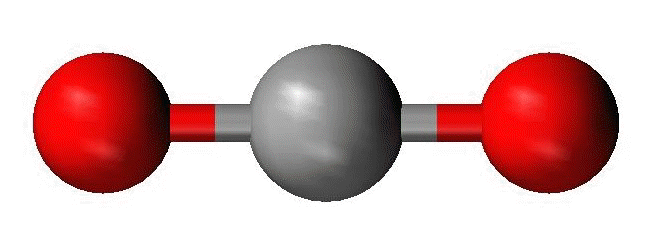Linear 180o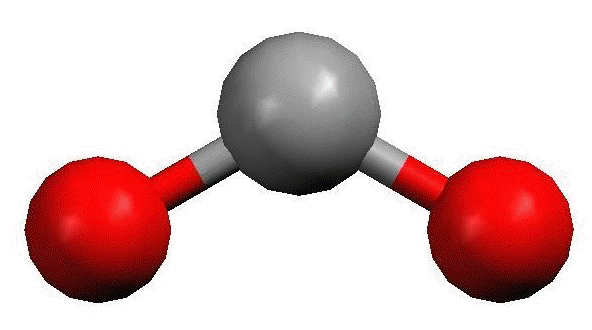Bent Less than 120 o (if there is one lone pair on the central atom)  Less than 109.5o (if there are two lone pairs on the central atom)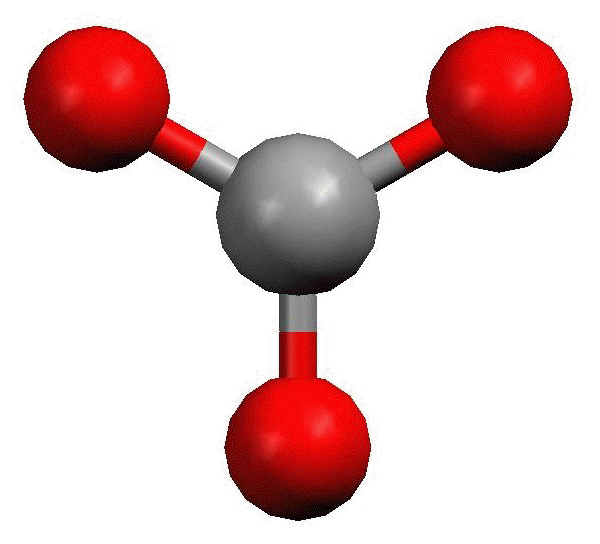Trigonal planar 120o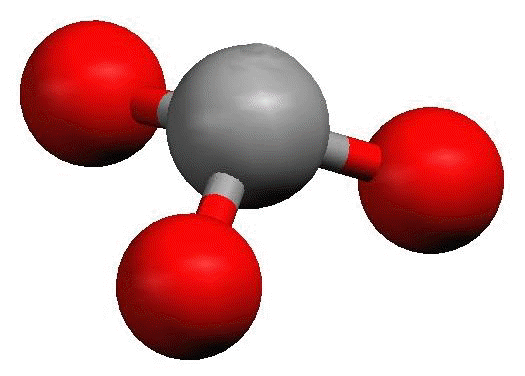Trigonal pyramid Less than 109.5o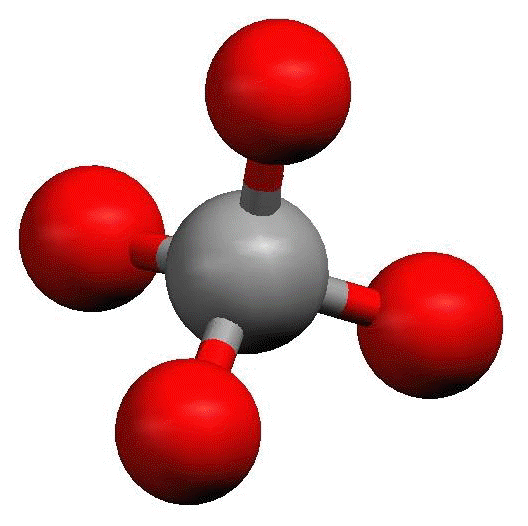Tetrahedral 109.5o

The difference in electronegativity of the atoms in a bond determine whether a bond is polar or nonpolar. Depending on the arrangement of the polar bonds in a three-dimensional molecular structure, the molecule can be classified as polar or nonpolar. In this experiment, students will also determine the polarity of the molecule based on the structure that they have drawn and modeled.

Section 2: Procedure

Part 1:  Drawing Lewis structures

 Calculate the total number of valence electrons of all atoms in the molecule or ion. For a polyatomic ion, add one electron for each negative charge, and subtract one for each positive charge. Look up and compare the electronegativities of the atoms. The least electronegative atom is the central atom, except hydrogen. Connect the other atoms to the central atom with a line (single bond). Add lone pairs so that each atom is surrounded by eight electrons (octet rule). Count 2 electrons for a single bond. Count the total number of electrons in the structure. If the structure contains more electrons than the total number of valence electrons calculated in step 1, form double bonds or triple bonds on the central atom as needed.

Part 2 Constructing molecular models, and determining molecular shapes

 Use your molecular model kit to construct a three-dimensional model of each of the molecules and polyatomic ions. Sketch a 3D representation of your model.    The following is a quick guide to using your molecular model kit:  Each colored ball corresponds to a specific atom. Refer to the color key provided in the kit.  Use the medium length gray links for single bonds.  Use the short white links for lone pairs.  Use the flexible long gray links for making double or triple bonds. Compare each model to the list of molecular shapes and identify the shape of the molecule. Determine the bond angle(s).

Part 3: Determining the polarity of the molecule

Using electronegativity values, evaluate the polarity of the bonds in each molecule. A bond is considered polar if the electronegativity difference between the bonded atoms is 0.5 to 0.9. A bond is considered nonpolar if the electronegativity difference between the bonded atoms is less than 0.5.

• A molecule will be nonpolar if it contains all nonpolar bonds, OR it contains polar bonds that are arranged in a symmetrical manner around the central atom.

• A molecule will be polar if it contains polar bonds that are arranged in a nonsymmetrical manner resulting from the presence of lone pairs on the central atom, or when there are different atoms surrounding the central atom

Section 3: Worksheet

1. CH4

 Total number of valence electrons: Lewis structure: 3D sketch: Molecular shape / bond angle          Polar or nonpolar?

1. CO2

 Total number of valence electrons: Lewis structure: 3D sketch: Molecular shape / bond angle          Polar or nonpolar?

1. NH3

 Total number of valence electrons: Lewis structure: 3D sketch: Molecular shape / bond angle          Polar or nonpolar?

Section 3: Worksheet (continued)

1. H2O

 Total number of valence electrons: Lewis structure: 3D sketch: Molecular shape / bond angle          Polar or nonpolar?

1. N2

 Total number of valence electrons: Lewis structure: 3D sketch: Molecular shape / bond angle          Polar or nonpolar?

1. SO2

 Total number of valence electrons: Lewis structure: 3D sketch: Molecular shape / bond angle          Polar or nonpolar?

Section 3: Worksheet (continued)

1. O2

 Total number of valence electrons: Lewis structure: 3D sketch: Molecular shape / bond angle          Polar or nonpolar?

1. O3 - use yellow ball for central atom

 Total number of valence electrons: Lewis structure: 3D sketch: Molecular shape / bond angle          Polar or nonpolar?

1. CO

 Total number of valence electrons: Lewis structure: 3D sketch: Molecular shape / bond angle          Polar or nonpolar?

Section 3: Worksheet (continued)

1. CO32-

 Total number of valence electrons: Lewis structure: 3D sketch: Molecular shape / bond angle          Polar or nonpolar?

1. NO3-

 Total number of valence electrons: Lewis structure: 3D sketch: Molecular shape / bond angle          Polar or nonpolar?

1. CF2Cl2

 Total number of valence electrons: Lewis structure: 3D sketch: Molecular shape / bond angle          Polar or nonpolar?

Post Lab Questions:

1. Which of the above molecules/ions has (have) more than one equivalent Lewis structures?

1. Which of the above molecular structures contain polar bonds, and yet the molecule is nonpolar? Explain briefly.

Experiment_621_Lewis Structures and Molecular Geometry_1_1_4 is shared under a CC BY license and was authored, remixed, and/or curated by LibreTexts.